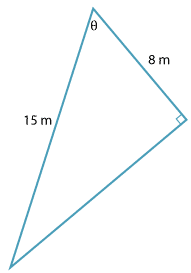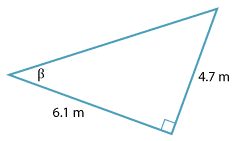#### Question 4Detailed description

The value of $$\theta$$ can be found by solving the equation:

 a $$\theta \ =\ \text{sin}^{−1}(\dfrac{8}{15})$$ $$\theta \ =\ \text{cos}^{−1}(\dfrac{8}{15})$$ $$\theta \ =\ \text{tan}^{−1}(\dfrac{8}{15})$$

#### Question 5Detailed description

The value of $$\beta$$ correct to the nearest degree is:

 a 52° 38° 50°